## Indicators

•Cited by SciELO
•Access statistics

•Cited by Google
•Similars in SciELO
•Similars in Google

## Print version ISSN 0121-1935

### rev. cienc. vol.18 no.2 Cali July/Dec. 2014

Lower Bound for the First Steklov Eigenvalue

Óscar Andrés Montaño Carreño
Departamento de Matemáticas, Universidad del Valle, Cali - Colombia
E-mail: oscar.montano@correounivalle.edu.co

Recceived: October 24, 2014
Accepted: December 18, 2014

Abstract

In this paper we find lower bounds for the first Steklov eigenvalue in Riemannian n-manifolds, n = 2, 3, with non-positive sectional curvature.

Keywords: First Steklov eigenvalue, sectional curvature.

1. Introduction

Let (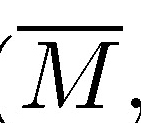,g) be a n-dimensional, compact, connected Riemannian manifold with smooth boundary ∂M. The operator L : C(∂M) → C(∂M) defined by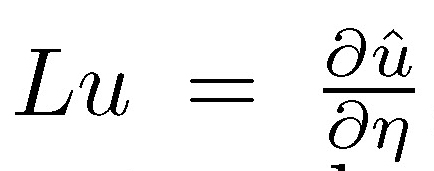where û is the harmonic extension over M of u and η is the unit outward normal to (∂M) is known as Dirichlet-Neumann operator. The first nonnule eigenvalue (υM) of L is called the first eigenvalue of the Steklov problem and it is variationaly characterized by: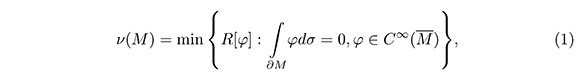where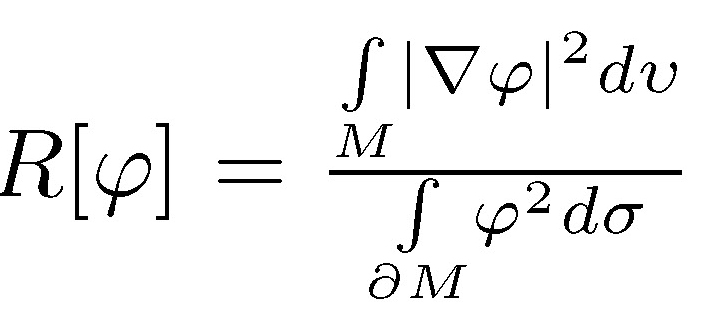is called Rayleigh quotient. For the Euclidian ball of the radio r > the first eigenvalue is υ 1/r with its own space generated by the coordinate functions. A function φ such taht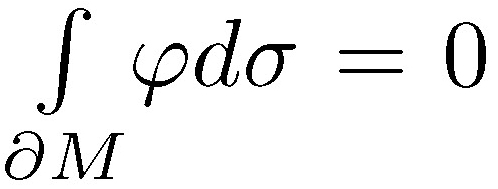space generated by the Starting from the variational characterization given by (1), if φ is a test function over M then υ (M) ≤ R |φ|.

In recent years, different authors, among them Weinstock (Weinstock, 1954), Kuttler and Sigillito (1968), Payne (1970), Escobar (1997; 1999; 2000), Wang and Xia (2009; 2010), Illias and Makloul (2011) and Montaño (2013a, 2013b, 2013c, 2014) among others have dealt with the problem of finding geometrical estimates for the first eigenvalue of Steklov.

In this article we find lower bounds for the first eigenvalue of Steklov in geodesic balls and simply connected domain of a Riemannian n-manifold; where n = 2,3, complete of non-positive sectiona curvature.

Our result is extended to Riemannian n-manifolds; where n = 2, 3, the estimate of Kutler-Sigillito for star-shaped domains of the plane (Kuttler & Sigillito, 1968).

2. Preliminaries

For this article, (M,g) will be a Riemannian n-manifold; where n = 2, 3, complete, simply connected and with non-positive sectional K curvature. Since M is complete, then for every pM the exponential function expp is defined over all TpM. Moreover, since K ≤ 0 over all M the Hadamard theorem (Docarmo, 1992)implies that expp : TpMM is a difeomorphism, that is, M is difeomorphic to nTpM, n = 2, 3.

Under the given hypothesis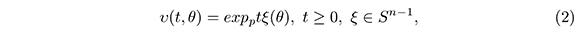it is a parameterization in geodesic coordinates for M.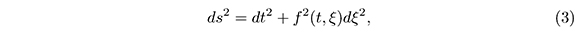where f(0,ξ) = 0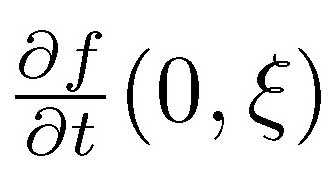= 1 and 2 is the metric of S1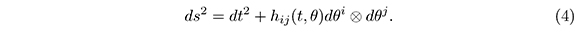For every t > 0 the boundary of the geodesic ball with center p and radio t, (p,t), is difeomorphic to S2 andn thus hij (t,θ) i >j is a metric over S2 The Uniformization theorem implies that the metric is conformally equivalent to the standard metric over S2 (Escobar 2000, p. 152). Therefore, we can assume that the metric over the 3-manifold is also in the form of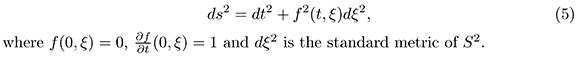Since the sectional curvature is non-positive, then the Bishop comparison theorem (Chavel, 1984) implies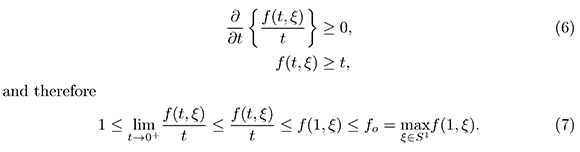3. Estimate for the First Eigenvalue over a Geodesic Ball

Theorem 3.1. If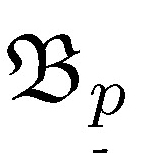M is a geodesic ball with a ratio 1 and center p ∈ M, then the first eigenvalue of Steklov satisfies the inequality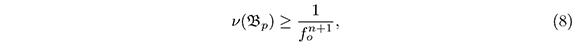where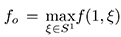The equality is achieved only ifisometric to the Euclidian ball of radio 1.

Proof. From (6) we have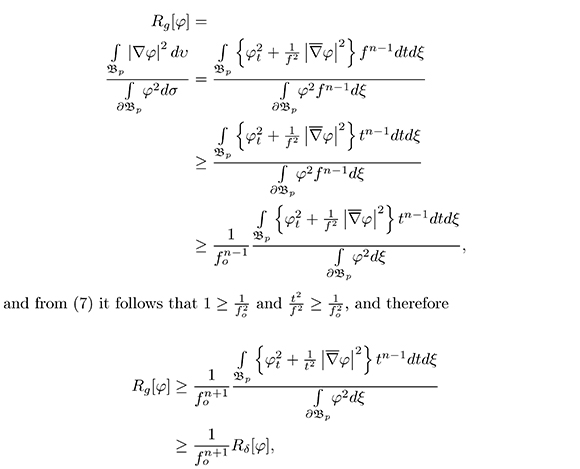where δ is the Euclidian metric and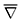is the gradient over Sn-1. If 1 - b is a eigenfunction for υ(, p) and choose the constant b such that ∂: = ∂1 - b serves as a test function for the first eigenvalue of the Euclidean ball υ(, p) = 1, thenThe equality is given only if f(t,ξ) = t, in suchis isometric to the Euclidian ball of radio 1.

From the Escobar comparison theorem (Escobar, 2000) and from our estimate, we have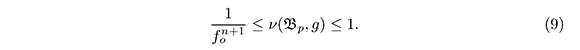4. Simply Connected Domains

In this section, D is a simply connected domain of TpM and Ω is olso a domaind M such that Ω = expp(D). υ(t,θ) = expptξ (θ) is a parametrization in geodesic cordinates of M, where ξ(θ) is a parametrization of Sn - 1. We suppose that the boundary of D is smooth adn it is given by ∂D = {Rξ : ξ ∈ Sn-1}, where R: Sn-1 is a strictly positive smooth function. In geodesic coordinates, the function F(t,θ) = t - R(θ) is such that ∂D and Ω are curves (surfaces) of level 0 of F in the parametrizations tξ(θ) and υ(t,θ) respectively. For this reason the unit normal vectors to each one of the boundaries are given by: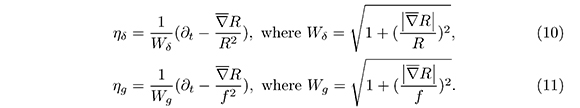From the previous identities, if we solve the equations cos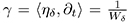and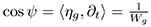we obtain: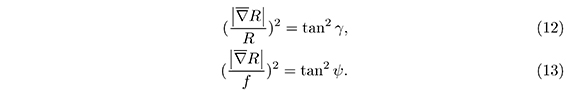From the inequality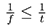it is deduced that over the boundaries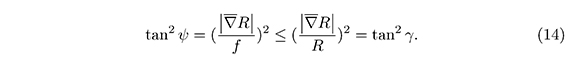4.1. Estimate for the Integral of the Squared Gradient Over Ω

Let us suppose that the angle γ satisfies the inequality: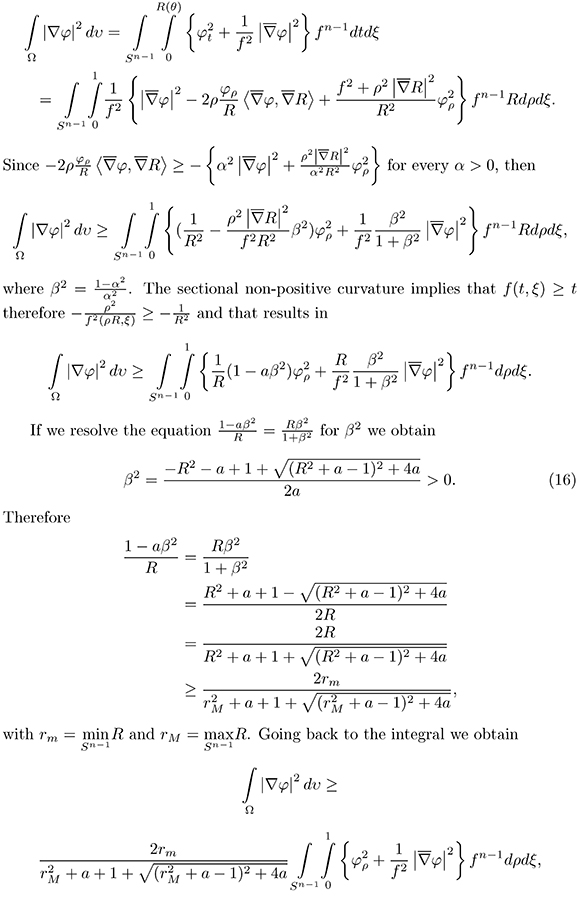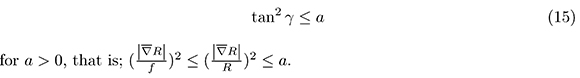getting the estimate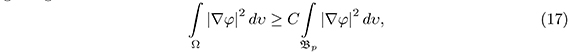whit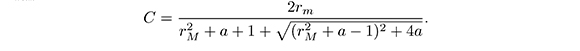4.2. Estimate for the Integral over the Boundary of φ2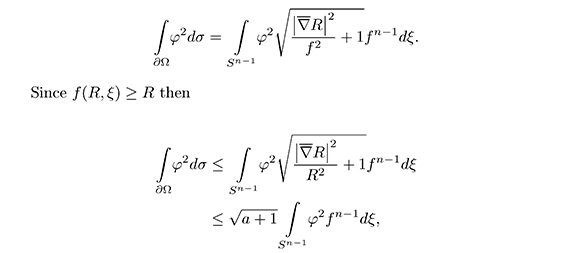getting the estimate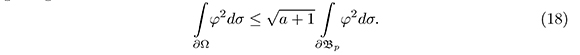4.3 Estimate for the rst eigenvalue of Steklov over Ω

From the estimates calculated in the previous sections (17), (18), we have the following estimate for the Rayleigh quotient:where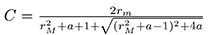andthe geodesic ball over M with center in p and radio 1.

If φ is a eigenfunction for the first eigenvalue over Ω and chose the constant b such that φ = φ1 -b erves as a test function for the first eigenvalue of the geodesic ball, thenReferences

Chavel, I. (1984). Eigenvalues In Riemannian Geometry. New York, USA: Academic Press, Inc.         [ Links ]

Chavel, I. (2005). Riemannian Geometry A Modern Introduction. Cambridge, USA: Cambridge University Press.         [ Links ]

Docarmo, M. P. (1992). Riemannian Geometry. Basilea, Suiza: Birkhauser.         [ Links ]

Escobar, J. F. (1997). The Geometry of the first Non-Zero Steklov Eigenvalue. Journal of Functional Analysis, 150, 544-556.         [ Links ]

Escobar, J. F. (1999). An Isoperimetric Inequality and the First Steklov Eigenvalue. Journal of Functional Analysis, 165, 101-116.         [ Links ]

Escobar, J. F. (2000). A comparison Theorem for the First non-zero Steklov Eigenvalue. Journal of Functional Analysis, 178, 143-155.         [ Links ]

Ilias, S., & Makhoul, O. (2011). A Reilly inequality for the first Steklov eigenvalue. Differential Geom. Appl., 5, 699 - 708.         [ Links ]

Kuttler, J. R., & Sigillito, V. G. (1968). Lower Bounds for Stekloff and free membrane eigenvalues. SIAM Review, 10, 368-370.         [ Links ]

Montaño, O. A. (2013a). The Stekloff problem for rotationally invariant metrics on the ball. Revista Colombiana de Matemáticas, 47(2), 181-190.         [ Links ]

Montaño, O. A. (2013b). Cota superior para el primer valor propio del problema de Steklov. Revista Integración, 31(1), 53-58.         [ Links ]

Montaño, O. A. (2013c). Cota superior para el primer valor propio de Steklov en el espacio euclídeo. Revista de Ciencias, 17(2), 95-103.         [ Links ]

Montaño, O. A. (2014). Métricas rotacionalmente invariantes y el problema de Steklov. Revista Integración, 32(2), 117-128.         [ Links ]

Payne, L. E. (1970). Some isoperimetric inequalities for harmonic functions. SIAM J. Math. Anal., 1, 354-359.         [ Links ]

Wang Q., & Xia, C. (2010). Inequalities for the Steklov Eigenvalues, arXiv:1006.1154.         [ Links ]

Wang, Q., & Xia, C. (2009). Sharp bounds for the first nonzero Steklov eigenvalues. J. Funct. Anal., 257, 2635-2654.         [ Links ]

Weinstock, R. (1954). Inequalities for a classical eigenvalue problem. J. Rational Mech. Anal., 3, 745-753.         [ Links ]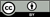Revista de Ciencias por Universidad del Valle se encuentra bajo una licencia Creative Commons Reconocimiento 4.0.All the contents of this journal, except where otherwise noted, is licensed under a Creative Commons Attribution License